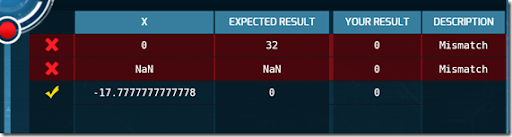## Thursday, February 12, 2015

### Creating Code Hunt Puzzles

As I mentioned the other day (see Learning From the Code Hunt Dashboard) I have been creating my own Code Hunt puzzles for use with my students. Earlier this week I spent some time at Microsoft Research in a workshop about Code Hunt. Among the things I learned was a few more things about creating puzzles. Specifically I learned more about how test cases are generated and how I can tune what test cases are created for my students to see. This can make puzzles harder or easier for students which is a good thing. Sometimes I want the test cases to point a little more clearly to a solution. I don’t want students too frustrated but at the same time I want some challenge to the puzzle. I learned a lot from the Code Hunt designer documentation which is available online here but I wanted to highlight a few things that others may find useful.

Let’s start with something simple. I created a puzzle where the correct solution is to convert a number from Celsius to Fahrenheit. Initially I created something that looked like this:

`   1: #level Cold`
`   2: #code C#`
`   3: using System;`
`   4: public class Program {`
`   5:     public static double Puzzle(double x) {`
`   6:         return 0.0;`
`   7:     }`
`   8: }`
`   9:  `
`  10: #secret C#`
`  11: using System;`
`  12: using Microsoft.Pex.Framework;`
`  13: public class Program {`
`  14:     public static double Puzzle(double x) {`
`  15:          return (9.0/5.0) * x + 32.0;`
`  16:     }`
`  17: }`

the problem for me is that the test data looks something like this:While the 0 to 32 is helpful I wanted a bit more of a hit. So I added a simple if with no action that forces 100 to 212 to run as test data. This makes the secret code more like this:

`   1: #secret C#`
`   2: using System;`
`   3: using Microsoft.Pex.Framework;`
`   4: public class Program {`
`   5:     public static double Puzzle(double x) {`
`   6:         if (x == 100);`
`   7:          return (9.0/5.0) * x + 32.0;`
`   8:     }`

The other issues I have had are with string based puzzles. While for some puzzles I want students to deal with the null case for some the early puzzles I want to remove that complexity. And in fact I may want to have a minimum number of characters in the string. For that there are some PexAssume methods we can use. I particularly like IsNotNullOrEmpty for avoiding having the null or empty string case. Though I could also use PexAssume.IsNotNull(s); to leave in the empty string case while removing the null string case. I can also use PexAssume.IsTrue to require that strings be at least a specific length.

`   1: PexAssume.IsNotNullOrEmpty(s);`
`   2: PexAssume.IsTrue(s.Length >= 3);`

To the computer a string is a string is a string and it doesn’t really care what characters is in it. So you can see strings like “/0/0/0” which can be a bit confusing and intimidating to beginners.

IT turns out that you can do some work in your secret code before you feed a value to a PexAssume.IsTrue method. That means that code like the following will make sure that the test data includes only the characters “a” though “z” are included in the test data.

`   1: Boolean b = true;`
`   2: for (int i = 0; i < s.Length;i++)`
`   3:     if (char.Parse(s.Substring(i, 1)) < 'a' || char.Parse(s.Substring(i, 1)) > 'z') b = false;`
`   4: PexAssume.IsTrue(b);`

I see a lot more potential in that sort of thing for the future.

I’ve been using PexAssume.IsNotNullOrEmpty for arrays as test data as well. I’ve got a zone that I have been using this semester but I am gradually adding puzzles as I learn more about Code Hunt. I’m willing to share my puzzles with others. Anyone else creating custom puzzle for their students?